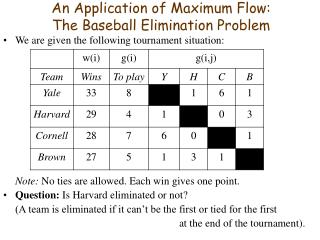# An Application of Maximum Flow: The Baseball Elimination Problem - PowerPoint PPT PresentationDownload PresentationAn Application of Maximum Flow: The Baseball Elimination Problem

An Application of Maximum Flow: The Baseball Elimination Problem
Download Presentation## An Application of Maximum Flow: The Baseball Elimination Problem

- - - - - - - - - - - - - - - - - - - - - - - - - - - E N D - - - - - - - - - - - - - - - - - - - - - - - - - - -
##### Presentation Transcript

1. An Application of Maximum Flow:The Baseball Elimination Problem • We are given the following tournament situation: Note: No ties are allowed. Each win gives one point. • Question: Is Harvard eliminated or not? (A team is eliminated if it can’t be the first or tied for the first at the end of the tournament).

2. The Baseball Elimination Problem: Preliminary Analysis • The maximum number of points Harvard can get is W = 29 + 4 = 33 (by winning all its games) • Suppose Harvard wins all its remaining games. It will not be eliminated if and only if • Brown has no more than u(B) = W-w(B) = 33-27 = 6 wins in the remaining games; • Cornell has no more than u(C) = W-w(C) = 33-28 = 5 wins in the remaining games; • Yale has no more than u(Y) = W-w(Y) = 33-33 = 0 wins in the remaining games. • Let Pbe the set of all the teams other than Harvard: P = {Y, C, B} • Let Q be the set of all possible pairs of P-teams: Q = { (Y,C), (Y,B), (C,B) } • The total number of games to be played between P-teams is G = 6+1+1 = 8 .

3. 6 Y Y,C 6 1 T C Y,B O 1 1 1 C,B B Solving the Baseball Elimination Problem via Maximum Flow • The baseball elimination problem can be solved by creating and solving a related instance of maximum flow problem: • Create a source node O (all the games originate here). • Create a node for each pair from Q; for each Q-node (i, j), add an arc from O to (i, j); the arc’s capacity is the number of games to be played between i and j. • Create a node for each team from P; for each Q-node (i, j), add arcs from (i ,j) to P-nodes i and j; cap( (i,j)i ) = cap( (i,j)j ) = cap( O (i,j) ) . • Create sink node T (the wins of the teams are recorded here). • Add an arc from any P-node j to T; the capacity of the arc is u(j) . 0 6 5 1 1 6

4. Y Y,C 5 5 5 1 T C Y,B O 2 1 1 1 C,B B Solving the Baseball Elimination Problem via Maximum Flow • Find the maximum flow from O to T in the resulting network. • If maximum flow value = G (total number of remaining games among P-teams) then Harvard still has chances to be number one, elseHarvard is eliminated. (that is, if all the games can be played so that teams Y, C, B get no more than u(Y), u(C), u(B) wins correspondingly, then Harvard still can be number one). • For our example, the bold red numbers on the arcs show the optimal flow values. Since the maximum flow value is 7 < 8 = G, Harvard is eliminated. 6 0 6 6 1 5 1 1 1 1 6 1

5. Y Y,C 5 5 5 1 T C Y,B O 2 1 1 1 C,B B Showing the elimination of Harvard using minimum-cut-based arguments • The O-side of the minimum cut is {O, (Y,C), Y, C} (the set of the nodes that are reachable from O via augmenting paths) • The team nodes on the O-side are Y and C. The number of games to be played between Y and C is 6. But the maximum number of total wins for Y and C, that allows Harvard to be number one, is 0+5 = 5. Thus, Harvard is eliminated. 6 0 Min cut 6 6 1 5 1 1 1 1 6 1

6. Showing the elimination of Harvard using minimum-cut-based arguments • Below is a different way to show the elimination of Harvard. The team nodes on the O-side are Y and C. The total number of wins between Y and C is (33 + 28) + 6 = 67. Then the average number of wins is 67 / 2 = 33.5 . This means that one of Y and C will certainly get ≥ 34points. So Harvard is eliminated with its maximum possible 33 points. • Generally, suppose we have teams 0, 1,…, n. If there is a set of teams R {1,…,n} such that (g(R) = total number of games to be played among R) then team 0 is eliminated. Claim: If team 0 is eliminated, then R = team nodes on the O-side of the minimum cut.